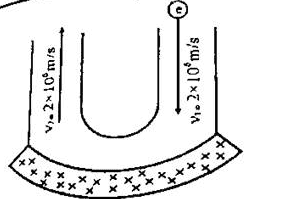# Reversing an electron's speed in a magnetic field

• Biker
In summary, the question is asking for the force exerted by a magnetic field on an electron in a given time period. Two different methods were used to calculate this force, one using the equation F=mv^2/r and the other using F=ma. The first method resulted in a force of 1.14 x 10^-15, while the second method resulted in a force of 7.28 x 10^-16. However, the second method is incorrect as it calculates the average force instead of the instantaneous force. The correct answer would depend on whether the question is asking for the net force or the centripetal force.

## Homework Statement

The following picture illustrates the passage of an electron a magnetic field. The magnetic field is trying to reverse the electron's velocity to the opposite direction. Calculate the force that the magnetic field is exerting on the electron in given time of ## t = 5 ~\text{x} ~10^{-9}##.. You are given the period of time which all of this happened## Homework Equations

## F = \frac{mv^2}{r} ##
## F = ma ##
## V = w r##
## w= \frac{2 \pi}/{T_Period}

## The Attempt at a Solution

I have used this way.
Magnetic field produces a force that is perpendicular to the velocity so it results in a circular motion
This reversing happen in half of a circle.
So
## w = \frac{\pi}{t} ##
Substitution of ## V = w r ## in ## F = \frac{mv^2}{r} ##
gives us
## F = m~ v ~w ##
Gives us the force
## F = 1.14~ \text{x}~ 10^{-15} ##

Now my friend used another way.
Which is ## F = m a ##
so ## F = m \frac{2v}{t} ##
which results in ## F = 7.28 ~\text{x} ~10^{-16} ##
However, this should be wrong because isn't this the net of all centripetal forces?
If I want the net of all of the magnetic forces I would use that equation

I translated the question, It isn't really clear of what it is asking for either the net force or the centripetal force. I just wanted to confirm the steps above

Last edited:
##2v## is the magnitude of the change in velocity for half a revolution of circular motion. Dividing this by the time of half a revolution gives the magnitude of the average acceleration for half a revolution. So your friend calculated the magnitude of the average force acting on the particle during half a revolution. The average force is different than the instantaneous force.

It might be helpful to consider a particle moving at constant speed in a complete circle. The change in velocity for a complete circle is zero. So the average acceleration is zero. Therefore, the average force for the complete circle is zero even though there is a nonzero centripetal force acting at each instant of time during the motion.

TSny said:
##2v## is the magnitude of the change in velocity for half a revolution of circular motion. Dividing this by the time of half a revolution gives the magnitude of the average acceleration for half a revolution. So your friend calculated the magnitude of the average force acting on the particle during half a revolution. The average force is different than the instantaneous force.

It might be helpful to consider a particle moving at constant speed in a complete circle. The change in velocity for a complete circle is zero. So the average acceleration is zero. Therefore, the average force for the complete circle is zero even though there is a nonzero centripetal force acting at each instant of time during the motion.
By average you mean net or sum?

I wasn't asking for how he got it XD. Just wanted to confirm the idea of "average" or net of the forces. And Yes, For a complete circle ## V-V = 0## so the acceleration for a complete circle is zero. We could draw multiple vectors and the net would be zero too.

Biker said:
By average you mean net or sum?
Average acceleration is defined to be ##a_{avg} = \frac{\vec{v}_f - \vec{v}_i}{\Delta t}##. This is what I meant by average acceleration. The average force is then mass time average acceleration.

I wasn't asking for how he got it XD. Just wanted to confirm the idea of "average" or net of the forces. And Yes, For a complete circle ## V-V = 0## so the acceleration for a complete circle is zero. We could draw multiple vectors and the net would be zero too.
OK. You are right, your friend's answer is wrong because he/she calculated the magnitude of the average force rather than the magnitude of the instantaneous force. (The question seems to be asking for the instantaneous force. However, I suppose that one could interpret the wording as asking for the average force. Then your friend would be correct!)